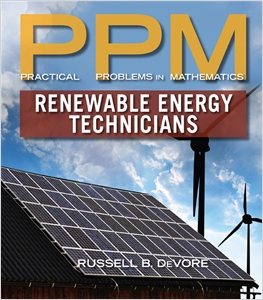I'm interested in (select all that apply)Practical Problems in Mathematics for Renewable Energy Technicians

ISBN 13: 9781285079332

ISBN 10: 1285079337

Author: Russell B. DeVore

Pages: 304

Published: 01/01/2016

Description
You can develop the essential quantitative skills you need to succeed in today's exciting, growing field of renewable energy, no matter what your current level of math skills, with DeVore's helpful PRACTICAL PROBLEMS IN MATHEMATICS FOR RENEWABLE ENERGY TECHNICIANS. This book presents important math concepts in short, easily understood units. The author clearly illustrates every concept with examples and practice problems drawn from the types of tasks that you will perform on the job every day. Loaded with helpful visual features and study aids, PRACTICAL PROBLEMS IN MATHEMATICS FOR RENEWABLE ENERGY TECHNICIANS puts key information at your fingertips with critical formula conversion charts, a glossary of the latest renewable energy terms, and plenty of exercise problems specifically designed to help you strengthen and develop confidence in your math skills.
Benefits
• LOGICAL ORGANIZATION BUILDS ON THE BASICS TO EQUIP STUDENTS FOR MORE ADVANCED CONCEPTS: The book begins with a clear introduction to the basics and gradually guides students toward more complex topics to challenge learners without overwhelming them.
• HELPFUL ILLUSTRATIONS CLARIFY CONCEPTS AND APPLICATIONS: Realistic drawings throughout this edition visually reinforce topics and help students more clearly understand abstract concepts as they see how concepts apply on the job.
• "PRACTICAL PROBLEMS" PRESENT A VARIETY OF ACTUAL CHALLENGES PROFESSIONALS FACE ON THE JOB: Throughout the text, examples and problem sets demonstrate how concepts apply to today's jobs in renewable energy. The text incorporates examples from five different types of renewable energy.
• HANDY REFERENCES CLARIFY IMPORTANT TERMINOLOGY AND KEY INFORMATION: A comprehensive glossary defines important terms, while helpful conversion charts present critical field information at a glance.
• ROBUST SUPPLEMENTS SAVE TIME IN PREPARATION AND GRADING: A comprehensive Instructor's Companion Website places essential resources at your fingertips, including a complete Solutions Manual, PowerPoint® presentation slides, and trusted test banks.
Author Bio
Russell B. DeVore
Russell B. DeVore [retired] was a Shift Technical Advisor and Simulator Instructor at the training center of a nuclear power plant for the Pennsylvania Power and Light Company. He is former Chair of the Arts & Sciences Division at Trident Technical College, and a former physics teacher at Bloomsburg University. Currently, he teaches on campus at a community college and online at a four-year state college. Dr. DeVore has worked in the energy and education fields for many years, contributing both academic and practical expertise to this book. He is also the author of PRACTICAL PROBLEMS IN MATHEMATICS FOR HEATING AND COOLING TECHNICIANS.SECTION 1: WHOLE NUMBERS.
2. Subtraction of Whole Numbers.
3. Multiplication of Whole Numbers.
4. Division of Whole Numbers.
5. Combined Operation with Whole Numbers.
SECTION II: COMMON FRACTIONS.
7. Subtraction of Common Fractions.
8. Multiplication of Common Fractions.
9. Division of Common Fractions.
10. Combined Operations with Common Fractions.
SECTION III: DECIMAL FRACTIONS.
12. Subtraction of Decimal Fractions.
13. Multiplication of Decimal Fractions.
14. Division of Decimal Fractions.
15. Decimal and Common Fraction Equivalents.
16. Combined Operations with Decimal Fractions.
SECTION IV: AVERAGES, RATIO AND PROPORTION.
17. Averages.
18. Ratio.
19. Proportion.
SECTION V: PERCENT, DISCOUNT, MARKUP AND EFFICIENCY.
20. Percentage.
21. Discount and Markup.
22. Efficiency.
Section VI: Direct Measure.
23. Equivalent Units of Temperature Measure.
24. Angular Measure.
25. Units of Length Measure.
26. Equivalent Units of Length Measure.
27. Equivalent Units of Additional Direct Measure.
SECTION VII: COMPUTED MEASURE.
28. Length Measure.
29. Area Measure.
30. Volume Measure.
31. Equivalent Units of Area and Volume Measure.
SECTION VIII: FORMULAS.
32. Electrical Relationships I Ohm's Law and Equivalent Resistances and Capacitances.
33. Electrical Relationships II Power.
34. Heat Transport.
35. Energy.
SECTION IX: TRIGONOMETRY.
36. Trigonometric Functions.
SECTION X: GRAPHS.
37. Graphs and Graphing.
SECTION XI: BILLS.
38. Estimates and Bills.
Appendix.